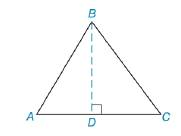Chapter 8.1, Problem 40E### Elementary Geometry for College St...

6th Edition
Daniel C. Alexander + 1 other
ISBN: 9781285195698

#### Solutions

Chapter
Section### Elementary Geometry for College St...

6th Edition
Daniel C. Alexander + 1 other
ISBN: 9781285195698
Textbook Problem
1 views

# Given: △ A B C , whose sides are 10 cm, 17 cm, and 21 cm. Find: a) BD, the length of the altitude to the 21-cm side b) The area of △ A B C , using the result from part (a)Exercises 39, 40

To determine

a)

To find:

The length of the altitude.

Explanation

Find the length of the altitude of the triangle is followed by the formula.

By Pythagorean theorem,

(BD)2+(DC)2=(BC)2

Calculation:

Given,

ABC, whose sides are 10 cm., 17 cm., and 21 cm., the length of the altitude BD to the 21-cm. side.

Let x be the length of DC and h be the length of the altitude BD.

For the right triangle BDC,

DC=x cm., BD=h cm., and BC=10 cm.

By Pythagorean theorem,

(BD)2+(DC)2=(BC)2

(h)2+(x)2=(10)2

h2+x2=100

h2+x2+(x2)=100+(x2)

h2+x2x2=100x2

h2=100x2

AD=(21x) cm, BD=h cm, and AB=17 cm

To determine

b)

To find:

The area of ABC, using the result from part (a).

### Still sussing out bartleby?

Check out a sample textbook solution.

See a sample solution

#### The Solution to Your Study Problems

Bartleby provides explanations to thousands of textbook problems written by our experts, many with advanced degrees!

Get Started

#### 12. Is if?

Mathematical Applications for the Management, Life, and Social Sciences

#### Evaluate 6 8 24 30

Study Guide for Stewart's Multivariable Calculus, 8th

#### Describe the general characteristics of the survey research design.

Research Methods for the Behavioral Sciences (MindTap Course List)